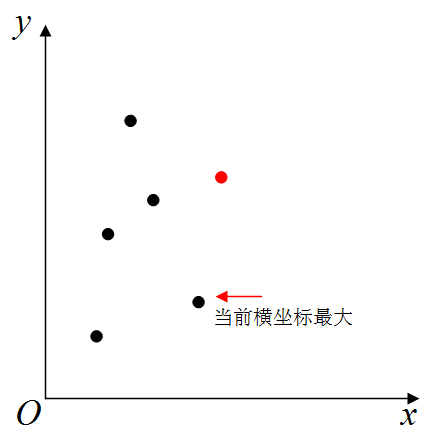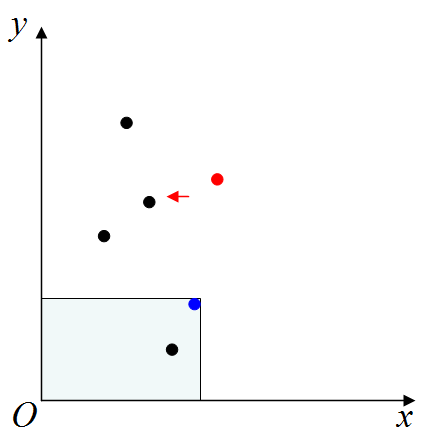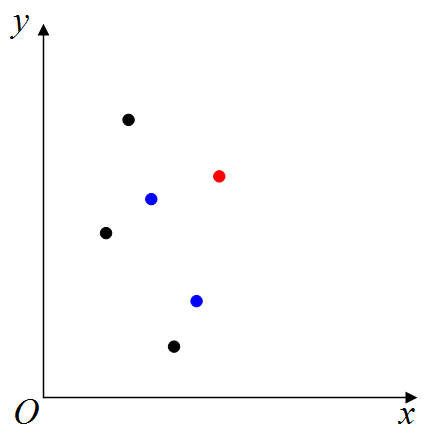# 洛谷P4066/bzoj1930 [SHOI2003]吃豆豆 题解【费用流】【最短路】

我估计现在为止洛谷上提交记录有一半都是我的。。。8
8 1
1 5
5 7
2 2
7 8
4 6
3 3
6 4

7

## 说明

N<=2000## 前言：

一开始费用流建模比较顺利，到最后发现RE了几个点以为是前向星边开小了，到最后开到MLE也跑不出来。造了个2000点的数据才发现是SPFA写挂了，然后同样的代码，别人的程序跑4,000,000次循环只用200ms，而我用了50s，卡到90分。。。在检查一下午之后发现是连边的问题，我建的模型有2×2000个点，稠密图下最多可能有8,000,000+(8e6)条边，参考了别人的代码才发现有很多边是不用连的。（连了既增加遍历的时间复杂度，又增加前向星的空间复杂度）2018.7.6目前为止提交记录里有一半都是我交的了## 题解：

这个题和传纸条有点像，不过传纸条是可以交叉，而这个题不能交叉。也就是每一个点只能有一个PACMAN通过，由于这个限制，我们可以联想到网络流的流量上限，豆豆作为费用，跑最大费用最大流。

如何控制每个点只被一个PACMAN吃掉，是我们要解决的一大问题。我们可以试着把一个点拆成一条边，使得这条边的流量为1，边权为1。也就是当且仅当这条边的两端都在最大流上时，费用才能算上，这就是建模的思路。也可以当一条边指向一个豆豆时，它的流量是1，不过这样会影响下面的优化，这种做法就是前面90分的做法。

我们回到题目，题目说PACMAN从原点出发，只能向右或向上，所以当两个点的斜率大于等于零或不存在时$((x1-x2)(y1-y2)\ge 0)$，两个点会连上一条边。如果这样做的话，那么当所有点之间的斜率都大于等于0，就成了一个稠密图，边数超过4e6，双向边加起来就有8e6了，我们就要想办法剪枝（这么多的边SPFA枚举都要花很长时间了），把有些点之间的联系用其他点当桥梁连接起来。

对于坐标系中一个点，它可以由横坐标非严格小于它，且纵坐标非严格小于它的点（在可行域中）转移。我们为了控制边数，只用连接与它最近的点。我们在可行域中首先找到横坐标最大（同等条件下纵坐标最大）的点，接着屏蔽掉以原点与这个点的连线为对角线的矩形，因为矩形中的点都可以或直接或间接地转移到这个右上角点来我们依次这样做下去，就会得到这两个蓝色点和红色点，从蓝点指向红点是一条边权为∞，费用为0的承接边不过，在某些情况下，下面剩的两个黑点直接走到红点是更优的解，这样我们只需要把之前拆的点之间重新建一条边，边权为∞，费用为0的承接边，表示不经过这个点的两点连线通过这个点连接到一起，与这个点无关。这样一来，与上面的拆点一起，每个点有了两条自环边，实则分成了两个点，它们之间有两条连线，一条是承接边，一条是费用边，即对费用增加有贡献的边

我们在这样的图上跑最大费用最大流，最终的流量大多数情况为2，答案为最大费用；当为1时说明一个PACMAN可以解决所有豆豆，此时答案为n。这样一来，所连接的边就约为2×2×2×n即8×n条，双向边最终前向星开100000就足够了。

需要注意一点，我们为了控制只有2个PACMAN，需要从源点1引出一条边指向一个源点2，边权为2，控制最大流不超过2。这样一来，就算有m个PACMAN，也可以通过控制这条边的边权来完成。

## Code：

//源点1为0，源点2为4002，汇点为4001，点i的入口为i，出口为2000+i。
#include<cstdio>
#include<cstring>
#include<algorithm>
struct edge
{
int n,v,w;
int nxt;
edge(int n,int v,int w,int nxt)
{
this->n=n;
this->v=v;
this->w=w;
this->nxt=nxt;
}
edge()
{
nxt=-1;
}
}e;
int head,cnt=-1;
void add(int from,int to,int v,int w)
{
e[++cnt].n=to;
e[cnt].v=v;
e[cnt].w=w;
e[cnt].nxt=head[from];
head[from]=cnt;
e[++cnt].n=from;
e[cnt].v=0;
e[cnt].w=-w;
e[cnt].nxt=head[to];
head[to]=cnt;
}
struct pnt
{
int x,y;
friend bool operator <(pnt a,pnt b)//把点排序
{
if(a.x!=b.x)
return a.x<b.x;
return a.y<b.y;
}
}p;
int pre;
int dis;
int q,l,r;
bool spfa()
{
bool used;//是否在队列中
memset(used,0,sizeof(used));
memset(dis,-0x3f,sizeof(dis));
l=r=0;
q[++r]=0;
dis=0;
used=true;
while(l<r)
{
int k=q[++l];
for(int i=head[k];~i;i=e[i].nxt)
{
int u=e[i].n;
if(e[i].v&&dis[u]<dis[k]+e[i].w)
{
dis[u]=dis[k]+e[i].w;
pre[u]=i;
if(!used[u])
{
used[u]=true;
q[++r]=u;
}
}
}
used[k]=false;
}
return dis>0;
}
int main()
{
int n;
scanf("%d",&n);
memset(head,-1,sizeof(head));
for(int i=1;i<=n;i++)
{
scanf("%d%d",&p[i].x,&p[i].y);
add(i,2000+i,1,1);
add(i,2000+i,2,0);
add(2000+i,4001,0x3fffffff,0);
add(4002,i,0x3fffffff,0);
}
std::sort(p+1,p+1+n);
add(0,4002,2,0);
for(int i=1;i<=n;i++)
{
int limy=0;
for(int j=i-1;j>=1;j--)
{
if(p[j].y>p[i].y||p[j].y<limy)
continue;
limy=std::max(limy,p[j].y);
add(2000+j,i,0x3fffffff,0);
}
}
pre=-1;
int sum=0;
while(spfa())
{
int t=pre;
sum+=dis;
while(~t)//增广
{
e[t].v--;
e[t^1].v++;
t=pre[e[t^1].n];
}
}
printf("%d\n",sum);
return 0;
}

### 说点什么Subscribe

/* */